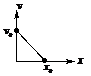The velocity displacement graph of a particle moving along a straight line is shownThe most suitable acceleration-displacement graph will be

# The velocity displacement graph of a particle moving along a straight line is shownThe most suitable acceleration-displacement graph will be

1. A
2. B
3. C
4. D

Register to Get Free Mock Test and Study Material

+91

Verify OTP Code (required)

### Solution:

The equation for the given v-x graph is  $\mathrm{v}=-\frac{{\mathrm{v}}_{0}}{{\mathrm{x}}_{0}}\mathrm{x}+{\mathrm{v}}_{0}$
Differentiating the above equation w.r.t. x, we get  $\frac{\mathrm{dv}}{\mathrm{dx}}=-\frac{{\mathrm{v}}_{0}}{{\mathrm{x}}_{0}}$
Multiplying the above equation in both sides by v, we get
$\mathrm{v}×\frac{\mathrm{dv}}{\mathrm{dx}}=-\frac{{\mathrm{v}}_{0}}{{\mathrm{x}}_{0}}×\mathrm{v}=-\frac{{\mathrm{v}}_{0}}{{\mathrm{x}}_{0}}\left[-\frac{{\mathrm{v}}_{0}}{{\mathrm{x}}_{0}}×+{\mathrm{v}}_{0}\right]\mathrm{From}\left(1\right)$$\mathrm{a}=\frac{{\mathrm{v}}_{0}^{2}}{{\mathrm{x}}_{0}^{2}}×-\frac{{\mathrm{v}}_{0}^{2}}{{\mathrm{x}}_{0}^{2}}...\left(2\right) \left[\because \mathrm{a}=\mathrm{v}\frac{\mathrm{dv}}{\mathrm{dx}}\right]$
On comparing the equation (ii) with equation of a straight line y= mx+c
We get $\mathrm{m}=\frac{{\mathrm{v}}_{0}^{2}}{{\mathrm{x}}_{0}^{2}}=+\mathrm{ve},\text{\hspace{0.17em}}\mathrm{i}.\mathrm{e}.\text{\hspace{0.17em}}\mathrm{tan\theta }=0,\mathrm{i}.\mathrm{e}.,\text{\hspace{0.17em}}\mathrm{\theta }$ is acute . Also $\mathrm{c}=-\frac{{\mathrm{v}}_{0}^{2}}{{\mathrm{c}}_{0}^{2}},\mathrm{i}.\mathrm{e}$ the intercept is negative. The above conditions are satisfied in graph (A)Register to Get Free Mock Test and Study Material

+91

Verify OTP Code (required)